# Motion of a Charged Particle in a Magnetic Field

Let us consider a charge q with velocityentering a region of space with a constant magnetic field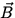. We assume that initiallyandare at right angles. The charge will experience the force of Eq.(1.2) which, by definition, is perpendicular to the velocity. Because of this, the force does no work on the charge (recall W = Fdcos= 0 if= 90 o ), and because W =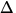K =(mv 2) the speed of the charge will not change. It turns out that the charge will move in a circular motion, with a (centripetal) acceleration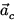directed toward the center of the circle, as illustrated in Fig. 1.3.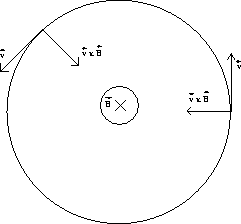For such a motion we have the following relation:

 F = qvB = m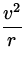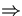r =. (3)

This behaviour of a charged particle in a magnetic field is the principle behind machines such as mass spectrometers, which can be used to measure the masses of charged particles by measuring their radii of curvature in a magnetic field.Next: Magnetic Force on a Up: Magnetism Previous: The Magnetic Field